# Wolfram Mathematica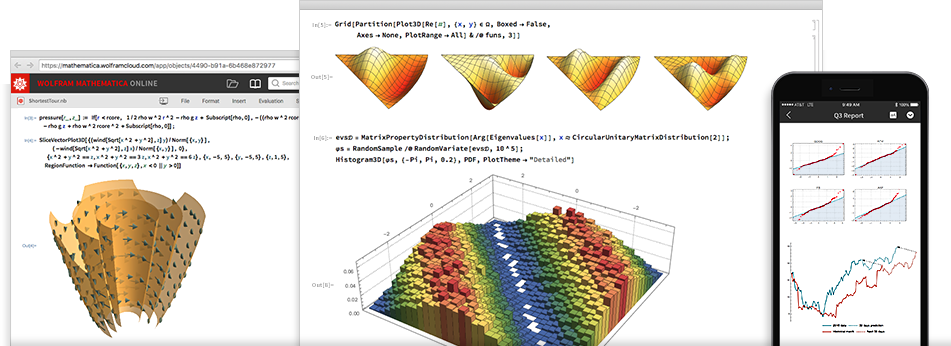Mathematica 在其三十年的开发历程中，在技术计算领域确立了最先进的技术，并为全球技术创新人员、教育工作者、学生和其他人士提供了最主要的计算环境。

Mathematica 以卓越的技术和简便的使用方法享誉全球，在此基础上，它提供了单个集成并且持续扩展的系统，涵盖了最广最深的技术计算功能，并可通过网页浏览器实现云端的完美访问，以及在所有现代桌面系统上的本地访问。## 现代技术计算的唯一选择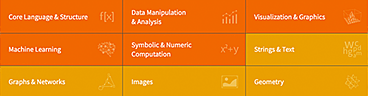### 一个全面集成的大型系统

Mathematica 具有涵盖所有技术计算领域的将近 6,000 个内置函数——所有这些都经过精心制作，使其完美地整合在 Mathematica 系统中。

### 不可想象的算法功能

Mathematica 在所有领域构建了前所未有的强大算法—许多算法都是使用 Wolfram 语言独特的开发方法和功能进行构建的

### 前所未有的高级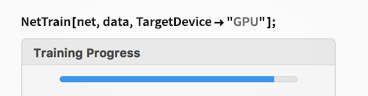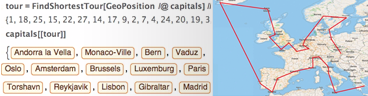### 易于使用的强大功能

Mathematica 凭借它的算法功能以及 Wolfram 语言的详细设计原理，创建了具有预测性建议、自然语言输入等的独特的并且易于使用的系统。

### 文档以及代码

Mathematica 使用 Wolfram 笔记本界面，使您可以快速整理包括文本、可运行代码、动态图形和用户界面等的丰富文档中的任何内容。

### 得到最美观的结果

Mathematica 使用最先进的计算美学和设计原理，为你呈现最美观的结果；立即创建最顶级的互动可视化效果和出版物质量级别的文档。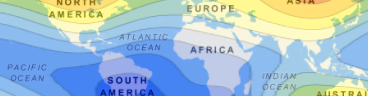### 即时现实数据

Mathematica 可以访问广博的 Wolfram 知识库，包括最实时的数千个领域的数据。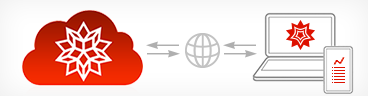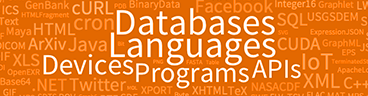### 完美的云端集成

Mathematica 目前已经完美地集成于云端系统中；可在统一强大的云端桌面混合环境中进行分享、云计算以及更多功能。

### 与任意内容连接

Mathematica 为与任意内容连接而构建：文件格式（180 多种）、其他语言、 Wolfram Data Drop、API、数据库、程序、物联网设备，甚至其自身分布等。

## 覆盖范围

Mathematica 基于具有突破性意义的 Wolfram 语言。

## 核心技术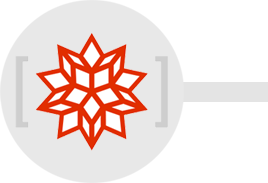## Mathematica 发展轨迹超过三十年的漫长历程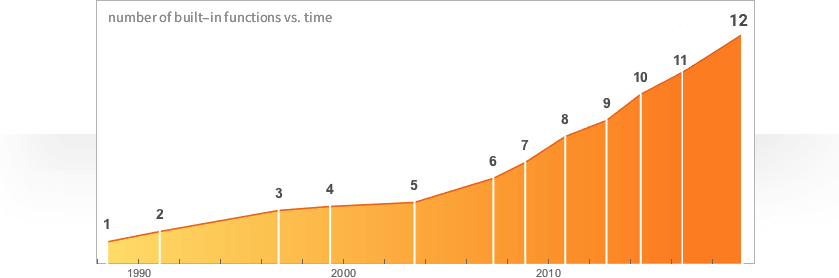### 创新速度越来越快

Mathematica 在三十年多的时间里遵从快速革新的发展轨迹，使得在每个阶段都构建了许多强大的新功能。

### 在每个版本中都有新思想

Mathematica 的各版本更新不仅仅是一般的软件更新；每个连续更新的版本都是在新方向上对计算模式的一次重大发展，并且引入了重要的新思路。

### 三十年多来的持续发展

Mathematica 忠实于它的核心准则和严肃的设计原理，持续发展并且集成了许多新功能和方法，而无需走回头路。## 在 Wolfram 产品世界中的 Mathematica

Mathematica 是 Wolfram 独创的旗帜产品；旨在为研发和教育工作提供技术计算平台。基于 Wolfram 语言，Mathematica 与其他核心 Wolfram 产品可以百分百兼容。